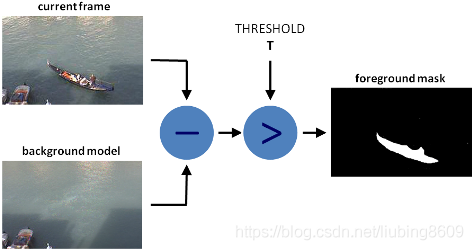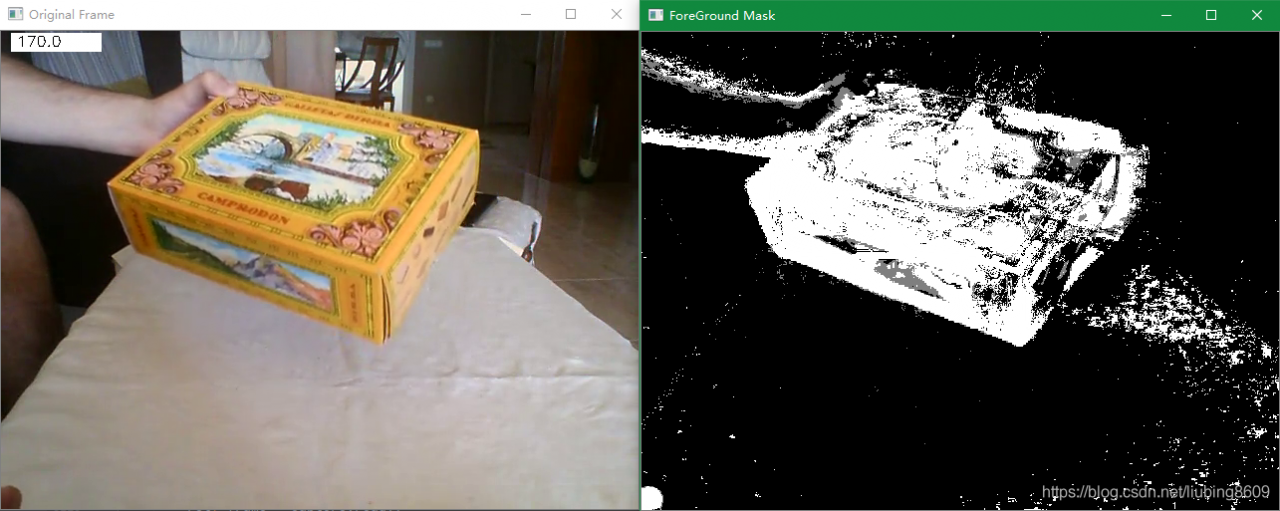# Python+OpenCV: How to Use Background Subtraction Methods

Python+OpenCV：How to Use Background Subtraction Methods
Background subtraction (BS) is a common and widely used technique for generating a foreground mask (namely, a binary image containing the pixels belonging to moving objects in the scene) by using static cameras. As the name suggests, BS calculates the foreground mask performing a subtraction between the current frame and a background model, containing the static part of the scene or, more in general, everything that can be considered as background given the characteristics of the observed scene.Background modeling consists of two main steps:

Background Initialization;Background Update.

In the first step, an initial model of the background is computed, while in the second step that model is updated in order to adapt to possible changes in the scene.
Background Subtraction in OpenCV

``````####################################################################################################
# 图像背景减法(Background Subtraction Methods)
def lmc_cv_background_subtraction():
"""
Function: Background Subtraction Methods.
""""

parser = argparse.ArgumentParser(description='This program shows how to use background subtraction methods\
provided by OpenCV. You can process both videos and images.')
parser.add_argument('--input', type=str, help='Path to a video or a sequence of image.',
default='D:/99-Research/Python/Image/box.mp4')
parser.add_argument('--algo', type=str, help='Background subtraction method (KNN, MOG2).', default='MOG2')
args = parser.parse_args()
# A lmc_cv::BackgroundSubtractor object will be used to generate the foreground mask.
if args.algo == 'MOG2':
back_sub = lmc_cv.createBackgroundSubtractorMOG2()
else:
back_sub = lmc_cv.createBackgroundSubtractorKNN()
# A lmc_cv::VideoCapture object is used to read the input video or input images sequence.
capture = lmc_cv.VideoCapture(lmc_cv.samples.findFileOrKeep(args.input))
if not capture.isOpened:
print('Unable to open: ' + args.input)
exit(0)
while True:
if frame is None:
break
# Every frame is used both for calculating the foreground mask and for updating the background.
# get the frame number and write it on the current frame
lmc_cv.rectangle(frame, (10, 2), (100, 20), (255, 255, 255), -1)
lmc_cv.putText(frame, str(capture.get(lmc_cv.CAP_PROP_POS_FRAMES)), (15, 15),
lmc_cv.FONT_HERSHEY_SIMPLEX, 0.5, (0, 0, 0))
# show the current frame and the ForeGround masks
lmc_cv.imshow('Original Frame', frame)